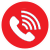# ISTQB – What is Boundary Value Analysis

## Boundary Value Analysis:

Boundary Value Analysis(BVA) comes from the understanding that programmers tend to make errors around the boundaries eg. when using  equal to(=), greater than, less than, greater than-equal to, less than-equal to or the number of times a loop should be run. Boundaries are quite common in any program. For example, consider the driving license application: age of applicant should be greater than or equal to 16 for driving license. In this example, the most likely errors would be around the boundaries :  ages equal to 15, 16, 17.

This idea of boundaries works well with equivalence partitioning because partitions must have boundaries.  Boundary Value Analysis is based on testing at the boundaries between partitions, and can be treated as an extension to the Equivalence Partitioning(EP).  Both EP and BVA work on range of numbers, values.

The rule is that we use the boundary value itself and one value(as close as you can get) on either side of the boundary. This is called ‘three-value BVA’ or the ‘full boundary value analysis’.

Eg. The boiling point of water is at 100 degrees Celsius, so the boundary values will be at 99, 100 and 101 degrees

Two-value Boundary value analysis: In this analysis, only the  boundary value and the invalid value are considered. So, for the boiling point example, only 2 values 100 and 101 will be considered.

Steps to identify the test cases:

1. Identify the Equivalence partitions – valid and invalid partitions. ( Please refer to the blog on Equivalence partitioning for more information).
2. Identify the boundaries for each of the partitions and write test cases for the boundaries identified using BVA.

Please note: The partitions should be tested separately from the boundaries – this means choosing partition values that are NOT boundary values.

Let us look at a sample BVA program taken from one of the ISTQB sample papers:

An employee has \$4000 of salary tax-free. The next \$1500 is taxed at 10%. The next \$28,000 is taxed at 22%. Any further amount is taxed at 40%. What are the valid equivalence partitions and boundary values for each partition?

Valid Equivalence Partitions: 1 to 4000,  4001 to 5500 ,  5501 to 33500,  >= 33501

2-value Boundary values for each valid partition:

• 0,1
• 4000, 4001
• 5500, 5501
• 33500, 33501

Full value BVA for each valid partition:

• 0,1 ,2
• 3900,4000, 4001
• 5499,5500, 5501
• 33500, 33501, 33502

This site uses Akismet to reduce spam. Learn how your comment data is processed.